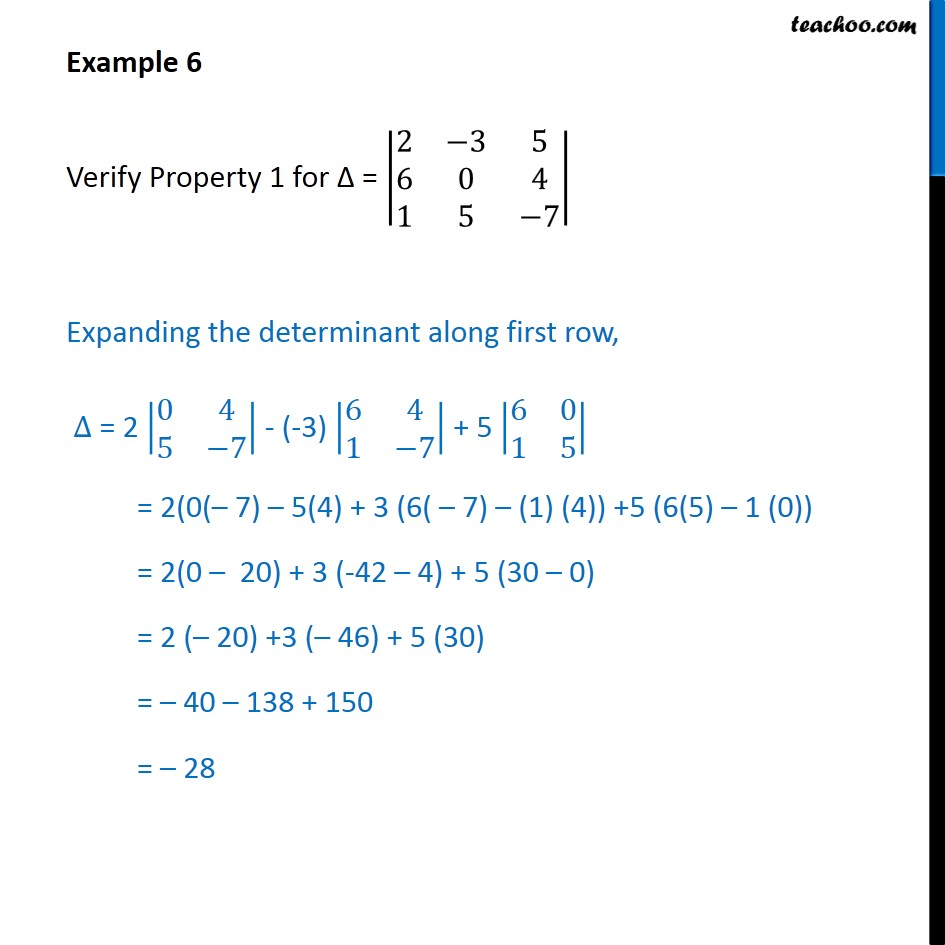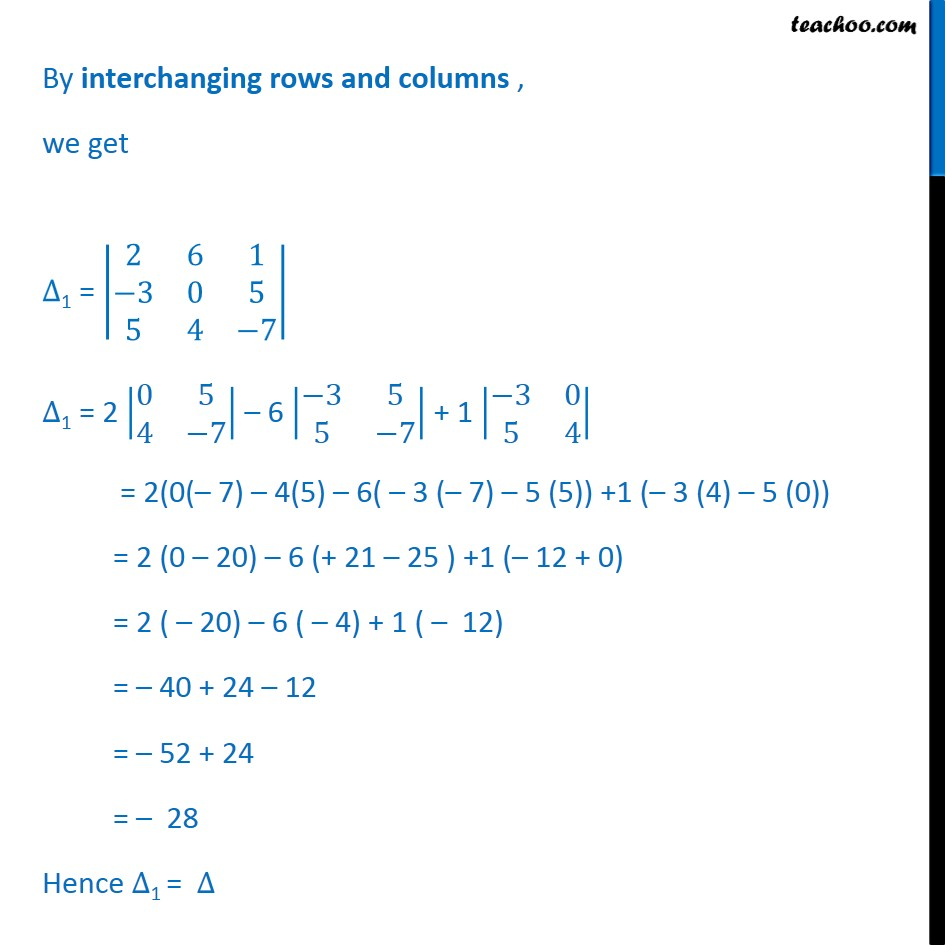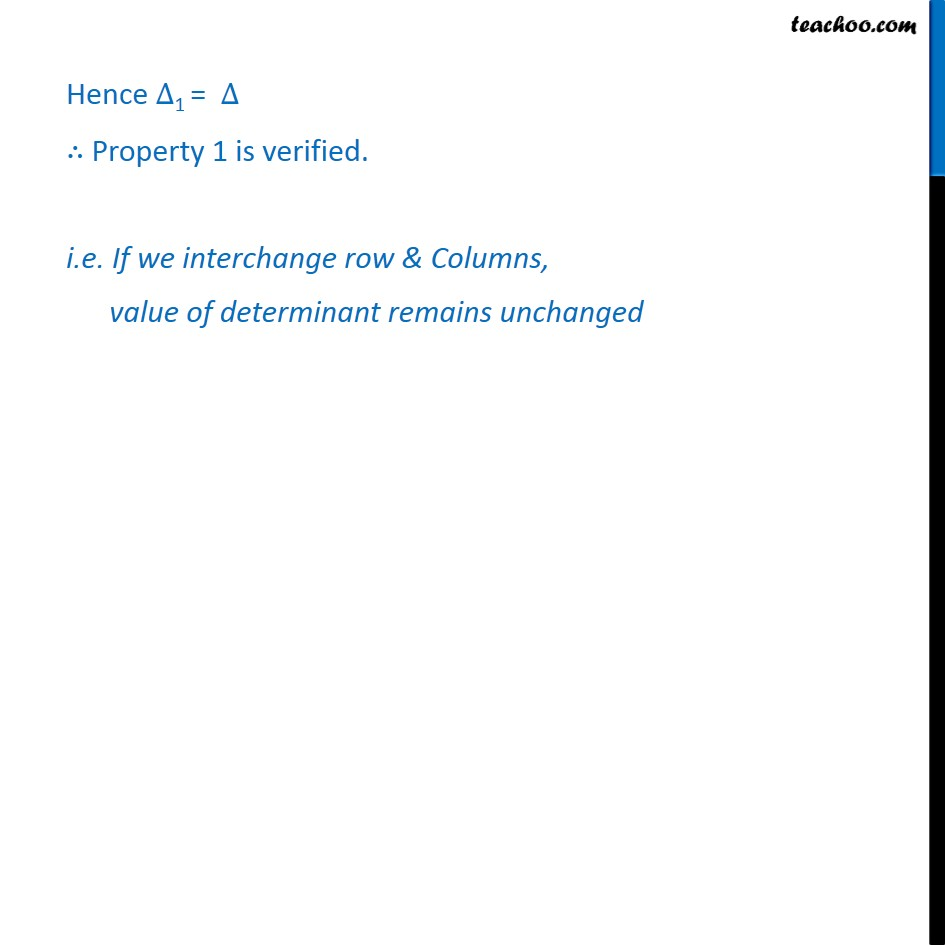Examples

Chapter 4 Class 12 Determinants
Serial order wiseLearn in your speed, with individual attention - Teachoo Maths 1-on-1 Class

### Transcript

Question 1 Verify Property 1 for = 2 3 5 6 0 4 1 5 7 Expanding the determinant along first row, = 2 0 4 5 7 - (-3) 6 4 1 7 + 5 6 0 1 5 = 2(0( 7) 5(4) + 3 (6( 7) (1) (4)) +5 (6(5) 1 (0)) = 2(0 20) + 3 (-42 4) + 5 (30 0) = 2 ( 20) +3 ( 46) + 5 (30) = 40 138 + 150 = 28 By interchanging rows and columns , we get 1 = 2 6 1 3 0 5 5 4 7 1 = 2 0 5 4 7 6 3 5 5 7 + 1 3 0 5 4 = 2(0( 7) 4(5) 6( 3 ( 7) 5 (5)) +1 ( 3 (4) 5 (0)) = 2 (0 20) 6 (+ 21 25 ) +1 ( 12 + 0) = 2 ( 20) 6 ( 4) + 1 ( 12) = 40 + 24 12 = 52 + 24 = 28 Hence 1 = Hence 1 = Property 1 is verified. i.e. If we interchange row & Columns, value of determinant remains unchanged